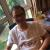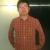# JSONObject类中getIntValue getLongValue等方法的设计建议

gtd 发布于 2012/12/12 16:05

```public Integer getInteger(String key) {
Object value = get(key);

return castToInt(value);
}

public int getIntValue(String key) {
Object value = get(key);

if (value == null) {
return 0;
}

return castToInt(value).intValue();
}```

```public static final Integer castToInt(Object value) {
if (value == null) {
return null;
}

if (value instanceof Integer) {
return (Integer) value;
}

if (value instanceof Number) {
return ((Number) value).intValue();
}

if (value instanceof String) {
String strVal = (String) value;
if (strVal.length() == 0) {
return null;
}

return Integer.parseInt(strVal);
}

throw new JSONException("can not cast to int, value : " + value);
}```

```public int getIntValue(String key, int defaultValue) {
Object value = get(key);

if (value == null) {
return defaultValue;
}
try {
return castToInt(value).intValue();
} catch(Exception e) {
return defaultValue;
}

}```

getInteger 返回包装类型的方法不抛异常，不能转成Integer直接返回null

getIntValue 返回原始数值类型的方法更也不用抛异常，返回默认值

00

0#### 引用来自“wenshao”的答案

```public long param(String name, long def_value) {
return NumberUtils.toLong(param(name), def_value);
}

public int param(String name, int def_value) {
return NumberUtils.toInt(param(name), def_value);
}

public byte param(String name, byte def_value) {
return (byte)NumberUtils.toInt(param(name), def_value);
}```

apache的NumberUtils中的toInt方法

```public static int toInt(String str, int defaultValue) {
if(str == null) {
return defaultValue;
}
try {
return Integer.parseInt(str);
} catch (NumberFormatException nfe) {
return defaultValue;
}
}```

0